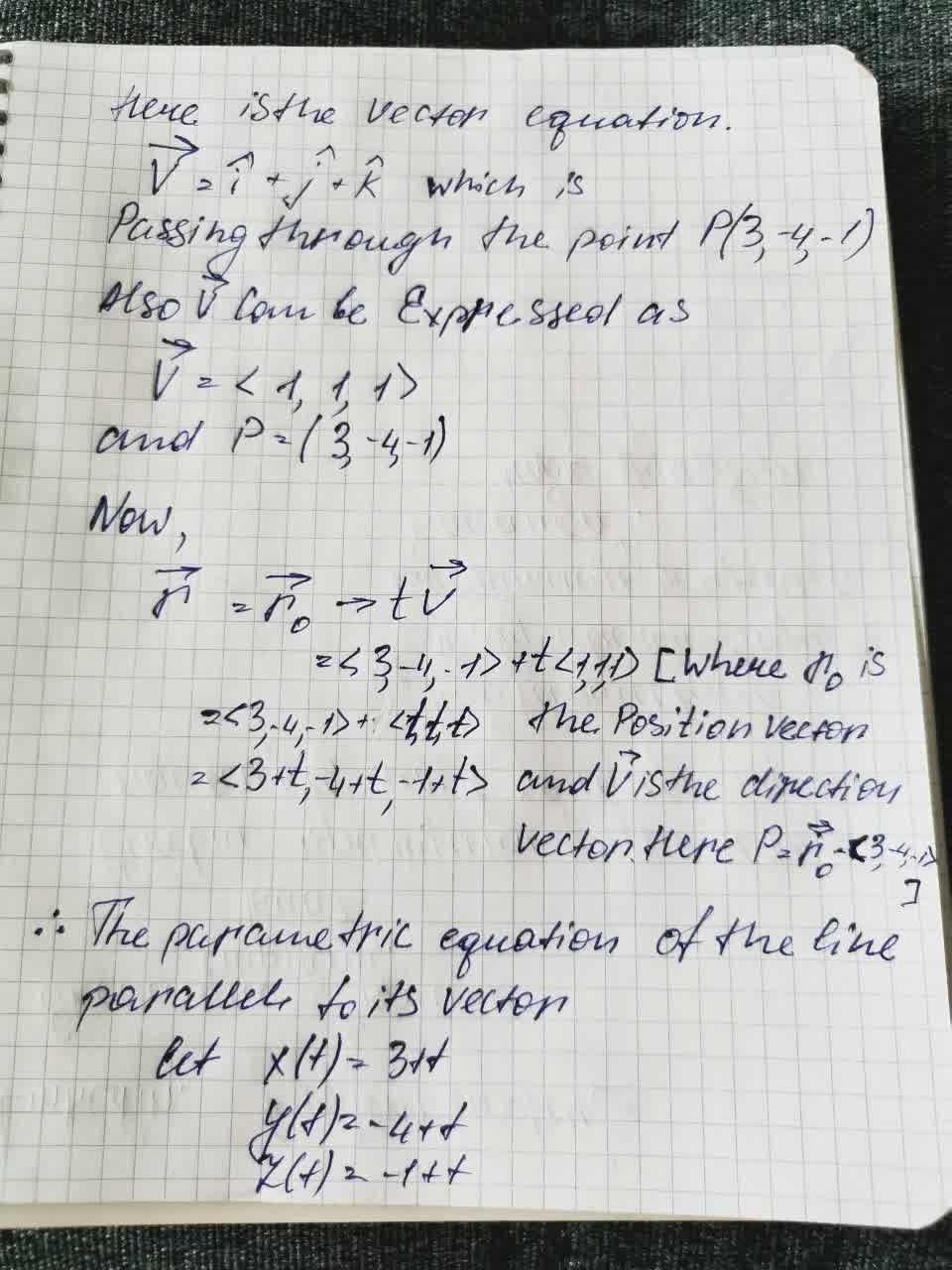# P(3,-4,-1) passing through point v=i+j+k.Write the parametric equation of the line parallel to its vectorIsa Trevino 2021-09-03 Answered

$$P(3,-4,-1)$$ passing through point $$v=i+j+k$$.
Write the parametric equation of the line parallel to its vector

• Questions are typically answered in as fast as 30 minutes

### Plainmath recommends

• Get a detailed answer even on the hardest topics.
• Ask an expert for a step-by-step guidance to learn to do it yourself.Nathaniel Kramer

To find the parametric equation